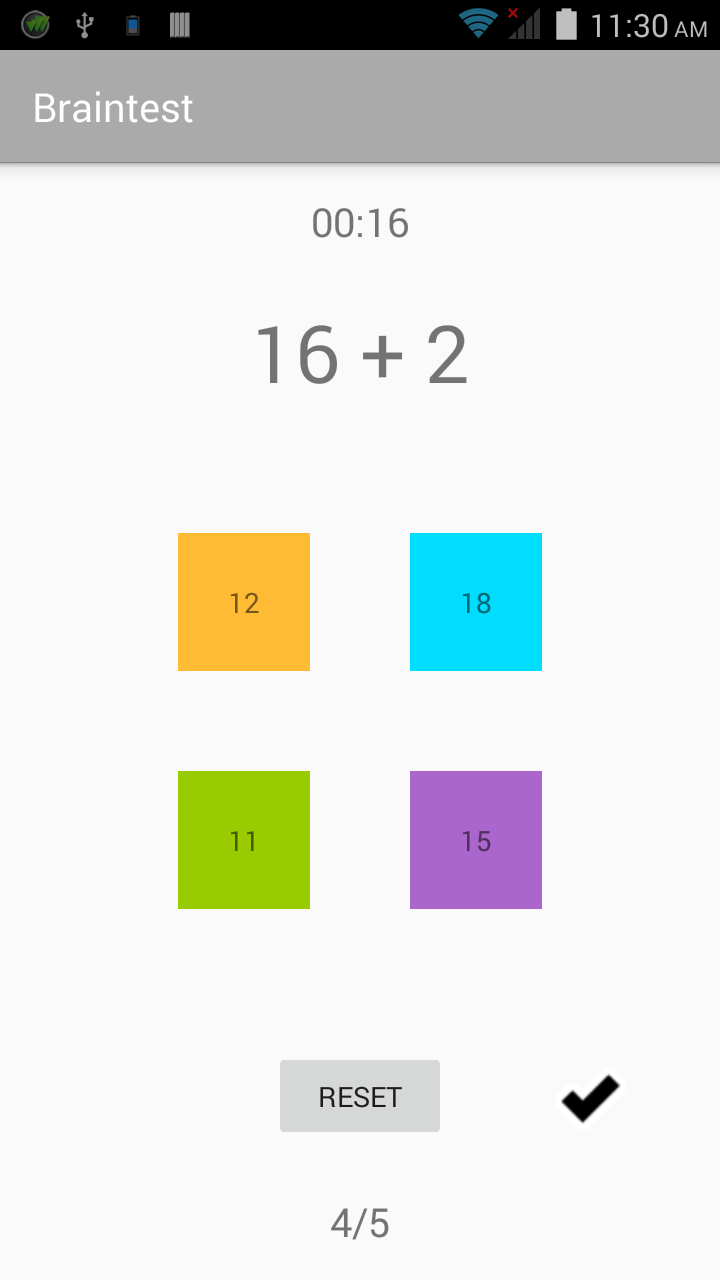For my continued education, I was required to make a brain teaser app which had several elements. Here is a download link if you want to try the finished product:

http://www.mediafire.com/file/xgmsnxqy58gdq8f/brain_test.apkThe whole thing hinged on this random number generator. I particularly struggled with this. I needed to have a question (x + y) and then have four random answers to pick from, only having one of which as the answer. At first I was working it from the beginning to the end: coming up with random x, and then adding random y, and then putting that into the text boxes randomly and making some new numbers. But, I eventually realized that I was working the problem the “long way around”.

Instead, I decided to make just one random answer, and split that into my question instead. This became much simpler. There are probably better ways to do this, but here is what I put together:

[CODE]
// And we need a random number generator.
public void randomNumber() {

// The actual number generator.
Random smallGen = new Random();

// To pick the tile threat is the answer.
// Remember: 0 is a number.

// Now to pick a number between 1 and 20.
// And assign that number to each tile.
randNumZero = smallGen.nextInt(19) + 1;
p0.setText(String.valueOf(randNumZero));

randNumOne = randNumZero + 6;
p1.setText(String.valueOf(randNumOne));

randNumTwo = randNumZero – 1;
p2.setText(String.valueOf(randNumTwo));

randNumThree = randNumZero + 3;
p3.setText(String.valueOf(randNumThree));

// And our random first number of our question.
// A number between 1 and 20. We will minus this from
// the answer to get the second number.
firstNum = smallGen.nextInt(19) + 1;

// Now to get the question.

secondNum = randNumZero – firstNum;

} else if (answerNum == 1) {

secondNum = randNumOne – firstNum;

} else if (answerNum == 2) {

secondNum = randNumTwo – firstNum;

} else {

secondNum = randNumThree – firstNum;

}

String fN = String.valueOf(firstNum);
String sN = String.valueOf(secondNum);

questionView.setText(fN + ” + ” + sN);

}
[/CODE]

Which worked great. Originally, I had created four random possible answers and then chose one at random as THE answer. However, I came to realize that sometimes the random possible answers could be the same as the random answer itself. That is when I changed randNumZero to be the only truly random number, the other randomNum objects are set additives and detraction from the actual random number. That makes them random to a point, since they are based from the random number.

After these possible answers are made, I then randomly choose one of them to be the answer, and from that minus a random number to get my two numbers for the question (the small minus random number and the derivative). Praise God! It even worked! This had an added benefit of allowing negative numbers, which really challenges the user’s math skills, since you only have 30 seconds to answer as many questions as possible.

Enjoy the math game!

Linux – keep it simple